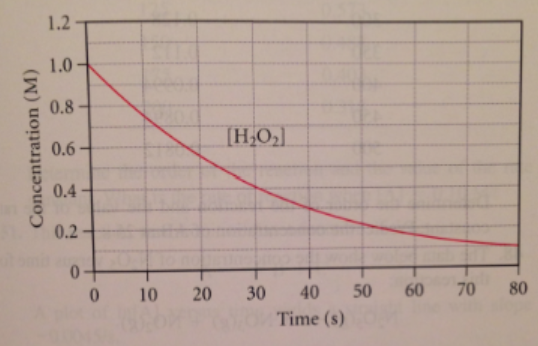# Problem: Consider the reaction:2 H2O2(aq) → 2 H2O(l) + O2(g)The graph below shows the concentration of H2O2 as a function of time.Use the graph to calculate each quantity:b.  The instantaneous rate of the reaction at 30 seconds.

###### FREE Expert Solution

We’re being asked for the instantaneous rate of the reaction at 30 seconds

An instantaneous reaction rate is the rate at a particular moment in the reaction and is usually determined graphically and can be calculated as:

Δ[  ] = change in concentration
Δt = change in time

82% (280 ratings)###### Problem Details

Consider the reaction:

2 H2O2(aq) → 2 H2O(l) + O2(g)

The graph below shows the concentration of H2O2 as a function of time.Use the graph to calculate each quantity:

b.  The instantaneous rate of the reaction at 30 seconds.

Frequently Asked Questions

What scientific concept do you need to know in order to solve this problem?

Our tutors have indicated that to solve this problem you will need to apply the Instantaneous Rate of Change concept. If you need more Instantaneous Rate of Change practice, you can also practice Instantaneous Rate of Change practice problems.